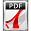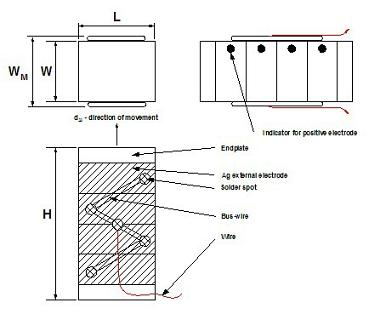# Micromechatronics, Inc. (MMech)

## www.mmech.com - Ph: 814-861-5688

#### Noliac Piezo ActuatorsDownload "Design Your Own Stack" as pdf

Noliac's standard stacks are designed with 1mm thick PZT endplates on top and bottom of the actuator, and n active single actuators.### Height

The total height of a SCMA can be estimated by the following equation:

H SCMA = n x H CMA + 2

Where: n = number of single actuators constituting the stack H CMA = height of the single actuators constituting the stack [mm]

Example:

Height of a a stack made of 14 CMAP7.

H CMAP7 = 2mm

H SCMA = 14 x 2 + 2 = 30mm

### Free displacement at maximum operating voltage

The maximal free displacement dL of a SCMA can be estimated by the following equation:

dL SCMA = 0,9 x n x dL CMA

Where:

n = number of CMA's constituting the SCMA

dL CMA = maximal displacement of the CMAs constituting the SCMA [m]

Example:

Displacement at 3KV/mm of a SCMA made of 14 CMAP7.

Displacement dL CMAP7 =3,1µm

Displacement dL =0,9 x 14 x 3,1E-6 = 39E-6m = 39µm

Note that those equations are only given as an indication. Please refer to Noliac for exact specifications.

All technical information of this document is not contractual and can be changed without prior notice